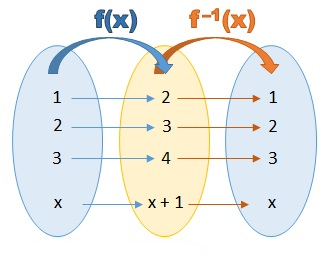# Functions Class 11 Mathematics Solutions | Exercise - 2.2## Chapter - 2  Functions

If you're studying mathematics in grade 11, you've probably come across the chapter on functions. In many textbooks, this chapter is listed as Chapter 2. Sometimes, when you're solving the problems in this chapter, you might face difficulties or get stuck. To assist you, I have the solutions for the class 11 function chapter.

In the function chapter, we will learn various methods to determine the domain and range of functions, as well as how to find the inverse of functions. This chapter consists of three exercises, each covering different subtopics related to functions. Before diving into the solutions section, let's gain a basic understanding of what functions are and how they work.

### Introduction

A function is a way to describe how one set of things relates to another set of things. It establishes a connection or relationship between the elements of the first set and the elements of the second set. In this relationship, each element from the first set corresponds to exactly one element in the second set, and no element is left unmatched.

We can represent this relationship using mathematical notations. For example, f(x) indicates that the function is named "f" and it takes an input value "x" from the first set, producing a corresponding output value. Alternatively, we can use the equation form y = f(x), where the function f(x) determines the value of "y" based on the input value "x". These notations help us express and work with functions in a concise and meaningful way.

### Inverse function

An inverse function, also known as an anti-function, is a function that can undo or reverse the action of another function. In simpler terms, if we have a function "f" that takes an input "x" and produces an output "y," then the inverse of "f" will take "y" as input and produce "x" as output.

To represent the inverse function, if the original function is denoted by 'f' or 'F', the inverse function is denoted by 'f⁻¹' or 'F⁻¹'. It's important not to confuse the '-1' notation used for the inverse function with exponents or reciprocals. In this context, the '-1' signifies the inverse relationship between the original function and its inverse.### Exercise - 2.2

Please note that this PDF focuses exclusively on Exercise 2.2. However, if you need solutions for other exercises, we've got you covered! Simply click the button above, and you'll find the solutions for those exercises as well. It's just a click away!

NoteScroll the PDF to view all Solution

You are not allowed to post this PDF in any website or social platform without permission.

## Is Class 11 Functions Note Helpful For Student ?

Absolutely! Our solutions for the Class 11 Mathematics Function Chapter are designed to greatly benefit students in their studies. Here are three key ways our solutions can help students excel in this subject:

### 1. Comprehensive Understanding

Our solutions provide students with a comprehensive understanding of the Function Chapter. Each step is explained in detail, guiding students through the problem-solving process. By following our solutions, students can grasp the underlying concepts and techniques required to tackle similar problems on their own.

### 2. Practice and Reinforcement

Practice is essential for mastering any subject, and mathematics is no exception. Our solutions offer a wealth of practice opportunities for students. By studying the solutions and attempting the problems independently, students can reinforce their learning, build confidence, and improve their problem-solving skills.

### 3. Exam Preparation

The solutions for the Function Chapter serve as an invaluable resource for exam preparation. Students can use these solutions as a reference to review concepts, learn alternative problem-solving approaches, and understand the expected format and presentation of solutions. By studying our solutions, students can gain insights into the types of questions that may appear in exams and develop strategies to approach them with confidence.

## How to secure good marks in Mathematics ?

As, you may know I'm also a student. Being a student is not so easy. You have to study different subjects simultaneously. From my point of view most of the student are weak in mathematics. You can take me as an example, I am also weak in mathematics. I also face problems while solving mathematics questions.

If you want to secure good marks in mathematics then you should practise them everyday. You should once revise all the exercise which are already taught in class. When you are solving maths problems, start from easy questions that you know already. If you do so then you won't get bored.

Maths is not only about practising, especially in grade 11 you to have the basic concept of the problem. When you get the main concept of the problem then you can easily any problems in which similar concept are applied.

When your teacher tries to make the concept clear by giving examples then all students tries to remember the same example but you should never do that. You can create your own formula which you won't forget later.

If you give proper time for your practise with proper technique then you can definitely score a good marks in your examination.

Disclaimer: This website is made for educational purposes. If you find any content that belongs to you then contact us through the contact form. We will remove that content from our as soon as possible.

If you have any queries then feel free to comment down.
No Comment